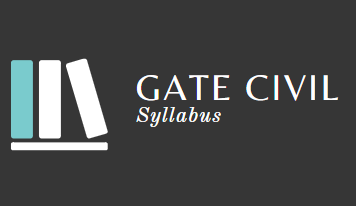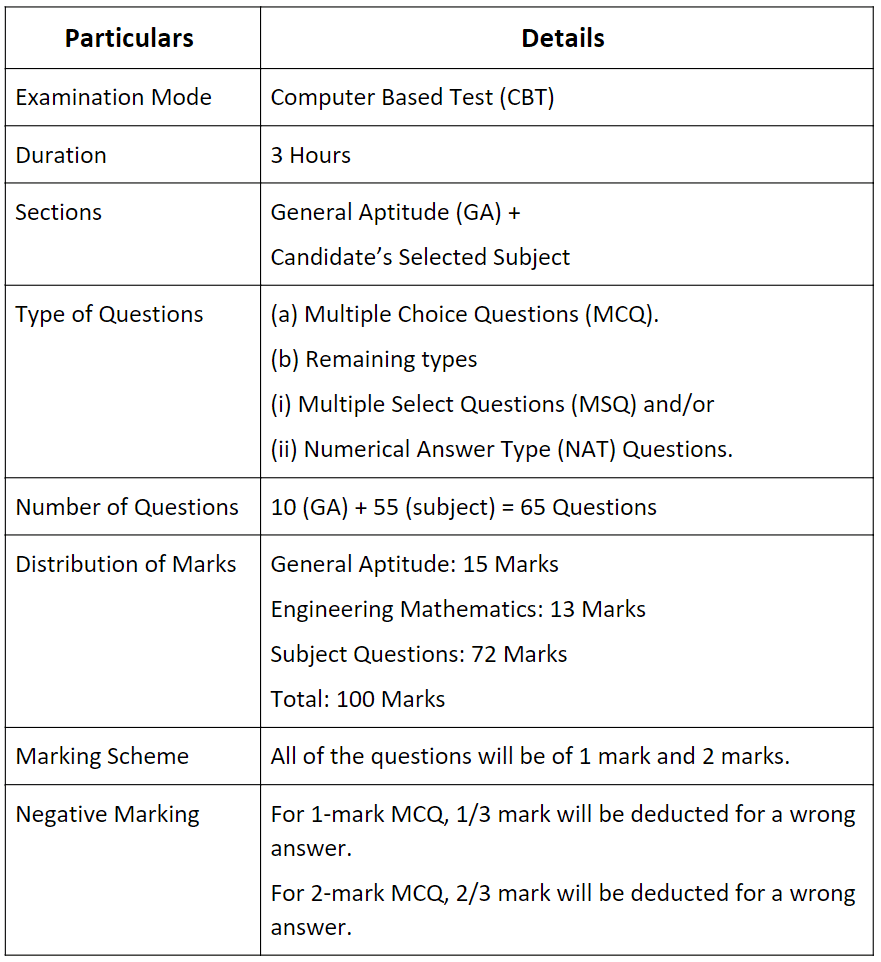Courses

# Syllabus - Civil Engineering, GATE Civil Engineering (CE) Notes | EduRev

## GATE : Syllabus - Civil Engineering, GATE Civil Engineering (CE) Notes | EduRev

The document Syllabus - Civil Engineering, GATE Civil Engineering (CE) Notes | EduRev is a part of the GATE Course GATE Past Year Papers for Practice (All Branches).
All you need of GATE at this link: GATE• Graduate Aptitude Test in Engineering (GATE) is a National-Level postgraduate engineering entrance exam for engineering graduate students. Usually, this is conducted by Indian Institute of Science, Bangalore and 7 other Indian Institute of Technology (IIT) located across India.
• It is conducted every year in the month of February and the next GATE entrance exam is expected to take place in the month of February 2022.
• This time it is IIT Kharagpur that is going to conduct the GATE 2022. IIT Kharagpur has released the syllabus for GATE Civil Engineering 2022.
• Candidates who are appearing for GATE from civil engineering discipline can refer the syllabus before they start their preparation.

Here is the list of topics and sub-topics for each subject that needs to be covered for the GATE 2022 Civil Engineering exam. Make sure the candidates get to know the following sections:

• General Aptitude
• Engineering Mathematics
• Structural Engineering
• Geotechnical Engineering
• Water Resources Engineering
• Environmental Engineering
• Transportation Engineering
• Geomatics Engineering

Candidates who are preparing for GATE 2022 Civil Engineering Exam can check the syllabus given below before you start the preparation.

Before getting into the details of GATE 2022 Civil Engineering Syllabus, let’s have an overview of GATE 2022 Exam Pattern for Civil Engineering.The three sections of the GATE paper are:
(i) General Aptitude
(ii) Engineering Mathematics
(iii) Subject-Specific Section

Let us now look into the detailed GATE syllabus for Civil Engineering.
Syllabus for General Aptitude (GA) (Common to all Papers)

1. Verbal Aptitude

• Basic English grammar: tenses, articles, adjectives, prepositions, conjunctions, verb-noun agreement, and other parts of speech.
• Basic vocabulary: words, idioms, and phrases in context.
• Narrative sequencing.

2. Quantitative Aptitude

• Data interpretation: data graphs (bar graphs, pie charts, and other graphs representing data), 2- and 3-dimensional plots, maps, and tables.
• Numerical computation and estimation: ratios, percentages, powers, exponents and logarithms, permutations and combinations, and series.
• Mensuration and geometry.
• Elementary statistics and probability.

3. Analytical Aptitude

• Logic: deduction and induction.
• Analogy
• Numerical relations and reasoning.

4. Spatial Aptitude

• Transformation of shapes: translation, rotation, scaling, mirroring, assembling, and grouping.
• Paper folding, cutting, and patterns in 2 and 3 dimensions.

Civil Engineering

1. Engineering Mathematics

• Linear Algebra: Matrix algebra; Systems of linear equations; Eigen values and Eigen vectors.
• Calculus: Functions of single variable; Limit, continuity and differentiability; Mean value theorems, local maxima and minima; Taylor series; Evaluation of definite and indefinite integrals, application of definite integral to obtain area and volume; Partial derivatives; Total derivative; Gradient, Divergence and Curl, Vector identities; Directional derivatives; Line, Surface and Volume integrals.
• Ordinary Differential Equation (ODE): First order (linear and non-linear) equations; higher order linear equations with constant coefficients; Euler-Cauchy equations; initial and boundary value problems.

• Partial Differential Equation (PDE): Fourier series; separation of variables; solutions of one- dimensional diffusion equation; first and second order one-dimensional wave equation and two-dimensional Laplace equation.

• Probability and Statistics: Sampling theorems; Conditional probability; Descriptive statistics - Mean, median, mode and standard deviation; Random Variables – Discrete and Continuous, Poisson and Normal Distribution; Linear regression.

• Numerical Methods: Error analysis. Numerical solutions of linear and non-linear algebraic equations; Newton’s and Lagrange polynomials; numerical differentiation; Integration by trapezoidal and Simpson’s rule; Single and multi-step methods for first order differential equations.

2. Structural Engineering

• Engineering Mechanics: System of forces, free-body diagrams, equilibrium equations; Internal forces in structures; Frictions and its applications; Centre of mass; Free Vibrations of undamped SDOF system.
• Solid Mechanics: Bending moment and shear force in statically determinate beams; Simple stress and strain relationships; Simple bending theory, flexural and shear stresses, shear centre; Uniform torsion, Transformation of stress; buckling of column, combined and direct bending stresses.
• Structural Analysis: Statically determinate and indeterminate structures by force/ energy methods; Method of superposition; Analysis of trusses, arches, beams, cables and frames; Displacement methods: Slope deflection and moment distribution methods; Influence lines; Stiffness and flexibility methods of structural analysis.
• Construction Materials & Management: Construction Materials: Structural Steel - Composition, material properties and behaviour; Concrete - Constituents, mix design, short-term and long-term properties. Construction Management: Types of construction projects; Project planning and network analysis - PERT and CPM; Cost estimation.
• Concrete Structures: Working stress and Limit state design concepts; Design of beams, slabs, columns; Bond and development length; Prestressed concrete beams.
• Steel Structures: Working stress and Limit state design concepts; Design of tension and compression members, beams and beam- columns, column bases; Connections - simple and eccentric, beam-column connections, plate girders and trusses; Concept of plastic analysis - beams and frames.

3. Geotechnical Engineering

• Soil Mechanics: Three-phase system and phase relationships, index properties; Unified and Indian standard soil classification system; Permeability - one dimensional flow, Seepage through soils – two - dimensional flow, flow nets, uplift pressure, piping, capillarity, seepage force; Principle of effective stress and quicksand condition; Compaction of soils; One- dimensional consolidation, time rate of consolidation; Shear Strength, Mohr’s circle, effective and total shear strength parameters, Stress-Strain characteristics of clays and sand; Stress paths.
• Foundation Engineering: Sub-surface investigations - Drilling bore holes, sampling, plate load test, standard penetration and cone penetration tests; Earth pressure theories - Rankine and Coulomb; Stability of slopes –Finite and infinite slopes, Bishop’s method; Stress distribution in soils – Boussinesq’s theory; Pressure bulbs, Shallow foundations – Terzaghi’s and Meyerhoff’s bearing capacity theories, effect of water table; Combined footing and raft foundation; Contact pressure; Settlement analysis in sands and clays; Deep foundations -dynamic and static formulae, Axial load capacity of piles in sands and clays, pile loadtest, pile under lateral loading, pile group efficiency, negative skin friction.

4. Water Resources Engineering

• Fluid Mechanics: Properties of fluids, fluid statics; Continuity, momentum and energy equations and their applications; Potential flow, Laminar and turbulent flow; Flow in pipes, pipe networks; Concept of boundary layer and its growth; Concept of lift and drag. Hydraulics: Forces on immersed bodies; Flow measurement in channels and pipes; Dimensional analysis and hydraulic similitude; Channel Hydraulics - Energy-depth relationships, specific energy, critical flow, hydraulic jump, uniform flow, gradually varied flow and water surface profiles.
• Hydrology: Hydrologic cycle, precipitation, evaporation, evapo-transpiration, watershed, infiltration, unit hydrographs, hydrograph analysis, reservoir capacity, flood estimation and routing, surface run-off models, ground water hydrology - steady state well hydraulics and aquifers; Application of Darcy’s Law.
• Irrigation: Types of irrigation systems and methods; Crop water requirements - Duty, delta, evapo-transpiration; Gravity Dams and Spillways; Lined and unlined canals, Design of weirs on permeable foundation; cross drainage structures.

5. Environmental Engineering

• Water and Waste Water Quality and Treatment: Basics of water quality standards – Physical, chemical and biological parameters; Water quality index; Unit processes and operations; Water requirement; Water distribution system; Drinking water treatment. Sewerage system design, quantity of domestic wastewater, primary and secondary treatment. Effluent discharge standards; Sludge disposal; Reuse of treated sewage for different applications.
• Air Pollution: Types of pollutants, their sources and impacts, air pollution control, air quality standards, Air quality Index and limits.
• Municipal Solid Wastes: Characteristics, generation, collection and transportation of solid wastes, engineered systems for solid waste management (reuse/ recycle, energy recovery, treatment and disposal).

6. Transportation Engineering

• Transportation Infrastructure: Geometric design of highways - cross-sectional elements, sight distances, horizontal and vertical alignments. Geometric design of railway Track – Speed and Cant. Concept of airport runway length, calculations and corrections; taxiway and exit taxiway design.
• Highway Pavements: Highway materials - desirable properties and tests; Desirable properties of bituminous paving mixes; Design factors for flexible and rigid pavements; Design of flexible and rigid pavement using IRC codes.
• Traffic Engineering: Traffic studies on flow and speed, peak hour factor, accident study, statistical analysis of traffic data; Microscopic and macroscopic parameters of traffic flow, fundamental relationships; Traffic signs; Signal design by Webster’s method; Types of intersections; Highway capacity.

7. Geomatics Engineering

• Principles of surveying; Errors and their adjustment.
• Maps - scale, coordinate system; Distance and angle measurement - Levelling and trigonometric levelling; Traversing and triangulation survey; Total station; Horizontal and vertical curves.
• Photogrammetry and Remote Sensing - Scale, flying height; Basics of remote sensing and GIS.
Offer running on EduRev: Apply code STAYHOME200 to get INR 200 off on our premium plan EduRev Infinity!

## GATE Past Year Papers for Practice (All Branches)

380 docs|127 tests

,

,

,

,

,

,

,

,

,

,

,

,

,

,

,

,

,

,

,

,

,

,

,

,

;